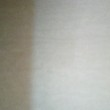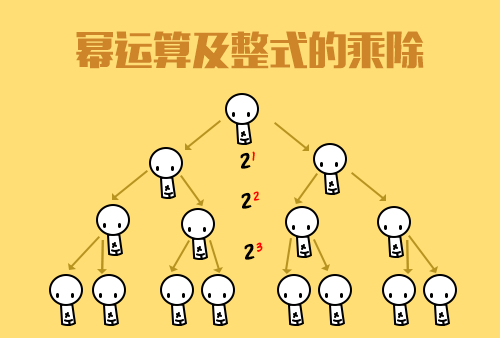| | |

||
APP端下载AndroidiPhone
￥0

|||

|
2197人点赞
1312046人已学习
|

3天无理由退款

3天无理由退款：退款将以超级币形式退至您的超级课堂学习账户，便于您重新选购其他课程。恶意退款将被冻结账号。

• 1、反比例函数的概念，当两个变量的乘积是一个固定的，不为$0$的常数时，他们就是反比例的关系
2、 反比例函数的解析式是：（$k$是常数，且$k\neq 0$），$k$也叫做比例系数
3、 反比例函数必须满足的三点：(1)、$k$是常数且$k$不为零。(2)、自变量$x$的指数是$-1$。(3)、解析式中除了比例系数$k$外没有其他常数。同时它的定义域是$x\neq 0$。
4、 求反比例函数解析式用待定系数法。注意多个函数在同一个式子中出现时，要用不同的字母来表示系数$k$
• 1、两支双曲线，无限接近$x$轴和$y$轴，但和坐标轴没有交点
2、 反比例函数的图象既是中心对称图形，又是轴对称图形
3、 对称中心是原点，当$k＞0$时对称轴是直线$y=x$，当$k$大于$0$时，图象位于一、三象限；当$k$小于$0$时对称轴是直线$y=-x$
4、 $k$对图像的影响：当$k$大于$0$时，图象位于一、三象限；当$k$小于$0$时，图象位于二、四象限。$\left | k \right |$决定了图象距离坐标轴的远近，$\left | k \right |$越大，图象离坐标轴越远
• 1、反比例函数的增减性：当$k> 2、 0$时，在每个象限内，$y$随$x$的增大而减小；当$k＜0$时，在每个象限内，$y$随$x$的增大而增大。凡是不强调“每一支曲线”的说法都是错的
3、 通过$x$的大小关系，判断$y$的大小关系时，如果几个$x$在$0$的同侧，也就是在同一支曲线上，那就只需根据$k$的正负判断$y$的大小；如果几个$x$在$0$的两侧，就需要你画图象，根据点的高低来判断$y$的大小
4、 根据$x$的范围，求分式的范围，像$\frac{K}{(Ax+B)}$（$A$、$B$、$k$是常数，且$A$和$k$都不为$0$）这种形式，只要把分母看成一个整体就可以了。记住，你一定要看图说话
• 1、正比例和反比例函数图像的位置关系，关键就是判断$k_{1}$$k_{2}$是否同号，当他们相交时，两个交点关于原点对称
2、 一次函数和反比例函数图像的位置关系，有相离、相切、相交三种，它们分别有$0$个、一个、两个交点。判断时需要联立方程组，确定一元二次方程根的情况，看看判别式的正负
3、 涉及到位置关系时，联立解析式。把几何问题，转化为代数问题，体现了数形结合的神奇简约
• 1、反比例函数中比例系数的几何意义，它决定了双曲线矩形的面积$\left | k \right |$
2、 同样也会有两个双曲线三角形，面积都固定为$\left | k \right |$的一半
3、 双曲线上演的一场百变大咖秀啦，各种变化，其实本质都是一样的，只要点在双曲线上，它就会遵循以上的规律
• 1、巧妙解决双曲线内嵌几何图形的问题。不论多么复杂的几何图形，只要找到图形中的关键点，设出点的坐标，再利用几何知识找到坐标的内在联系，同时利用解析式加以限定。就可以通过列方程解出所设点的坐标
2、 记住一个小结论，同一条双曲线上，如果$A$的横坐标是$B$的$n$倍，那么$A$的纵坐标就是$B$的$n$分之一

• 1超级学员816150
• 2超级学员841627
• 312345
• 4超级学员875743
• 5超级学员882384
• 6超级学员882733
• 7超级学员884340
• 812ABC
• 9超级学员887791
• 10超级学员888136767546人在学
￥ 330 ￥ 1851306150人在学
￥ 210 ￥ 8756964人在学
￥ 330 ￥ 220755534人在学
￥ 270 ￥ 175

• 0
• 点击分享有好礼
•app端下载关注微信号